# 3 years savings

Simple interest problem. Tereza has deposited CZK 100,000 in the bank with an annual interest rate of 1.5%. Interest is added each year and left aside. How many CZK (crowns) will have total after 3 years?

x =  104500 Kc

### Step-by-step explanation: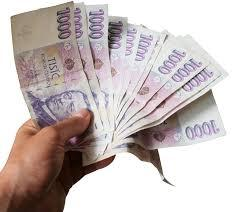Did you find an error or inaccuracy? Feel free to write us. Thank you!Tips to related online calculators
Do you want to convert time units like minutes to seconds?

## Related math problems and questions:

• Compound interestCompound interest: Clara deposited CZK 100,000 in the bank with an annual interest rate of 1.5%. Both money and interest remain deposited in the bank. How many CZK will be in the bank after 3 years?
• Bank account CZWe will deposit CZK 6,600 into the bank for 1 year with an interest rate of 2.5%. The interest tax is 15%. How many crowns will we receive after one year?
• Combined interest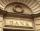Combined interest: Carol has deposited CZK 100,000 in the bank with an annual interest rate of 1.5%. The money was put into the account 5.5.2016 and withdraw them all before Christmas 20.12.2016. How much money did she withdraw?
• DepositOh I total of $15,000 deposited into simple interest accounts the annual simple interest rate on one account at 6% the annual simple interest rate on the second account at 7% how much should be invested in each account so that the total interest earned is • Deposit is pesosSally deposits Php.22,000 in her savings account. If the bank pays 1.5% interest per year, how much will she receive at the end of the year? • BankPaul put 10000 in the bank for 6 years. Calculate how much you will have in the bank if he not pick earned interest or change deposit conditions. The annual interest rate is 3.5%, and the tax on interest is 10%. • SavingsThe depositor regularly wants to invest the same amount of money in the financial institution at the beginning of the year and wants to save 10,000 euros at the end of the tenth year. What amount should he deposit if the annual interest rate for the annua • DepositIf you deposit 719 euros at the beginning of each year, how much money we have at 1.3% (compound) interest after 9 years? • Profitable bank deposit 2012Calculate the value of what money lose creditor with a deposit € 9500 for 4 years if the entire duration is interest 2.6% p.a. and tax on interest is 19%. Annual inflation is 3.7% (Calculate what you will lose if you leave money lying idle at negative int • Compound interest 3After 8 years, what is the total amount of a compound interest investment of$25,000 at 3% interest, compounded quarterly? (interest is now dream - in the year 2019)
• ShuyenShuyen wanted to save some money. She deposited Php.300 in a bank which pays 0.5% interest per annum. After nine months, she needed the money to buy some gifts. How much will she be able to get if he withdraws all her money from the bank?
• Repay, interest, loanRamchacha takes a loan amount of 240000 from a bank for constructing a house at the rate of simple interest of 12% per annum. After 1 yr. of taking the loan he rents the house at the rate of 5200 per month. Determine the number of years he would take to r
• Annual interest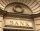A loan of 10 000 euro is to be repaid in annual payments over 10 years. Assuming a fixed 10% annual interest rate compounded annually, calculate: (a) the amount of each annual repayment (b) the total interest paid.
• Compound interest 4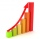3600 dollars is placed in an account with an annual interest rate of 9%. How much will be in the account after 25 years, to the nearest cent?
• Account operationsMy savings of php 90,000 in a bank earns 6% interest in a year. If i will deposit additional php 10,000 at the end of 6 months, how much money will be left if i withdraw php 25,000 after a year?
• InflationOnce upon a time, tsar owned a money printer and printed and printed. The result of printing money prices went up,in the first year 3.9 %, in the second 6%, in the third 4.7% and in the fourth 5.5%. Then tsar was failed in election. Calculate the average
• Two years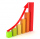Roy deposited 50,000.00 into his account paying 4% annual interest compounded semi annually. How much is the interest after 2 years?